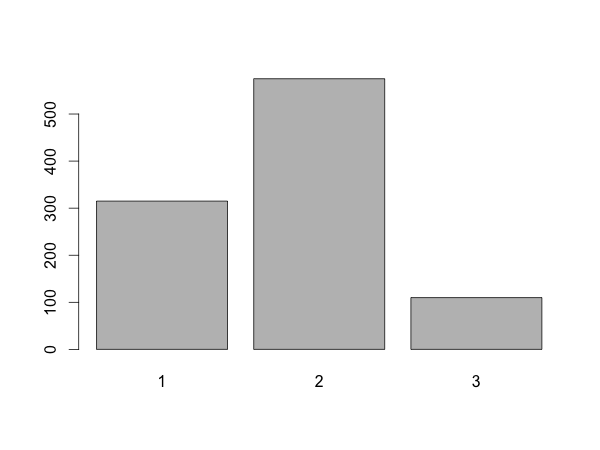```print(sample(1:3))
print(sample(1:3, size=3, replace=FALSE))  # same as previous line
print(sample(c(2,5,3), size=4, replace=TRUE)
print(sample(1:2, size=10, prob=c(1,3), replace=TRUE))
```
``` 3 1 2
 2 1 3
 2 5 2 2
 2 2 2 1 1 2 2 2 1 2
```

## Overview

By default sample() randomly reorders the elements passed as the first argument. This means that the default size is the size of the passed array. replace=TRUE makes sure that no element occurs twice.

The last line uses a weighed random distribution instead of a uniform one. One out of four numbers are 1, the out of four are 3.

## Arguments

### size

This is the size of the returned list. If replace is disabled size must be no bigger than the length of the first argument.

### replace

If this is true a sample may contain an element several times while another element might not occur at all.

```print(sample(c(2,5,3), size=3, replace=FALSE))
print(sample(c(2,5,3), size=3, replace=TRUE))
```
``` 2 3 5
 2 3 3
```

Allowing some elements to occur more than once lets you get a sample longer than the first argument.

### prob

```barplot(table(sample(1:3, size=1000, replace=TRUE, prob=c(.30,.60,.10))))
```The prob=c(.30,.60,.10) cause 30% ones, 60% twos and 10% threes. The numbers don't have to add up to 1 - they don't in the example at the top of the page.

Table sums up the individual items in the 1000-element list.